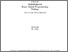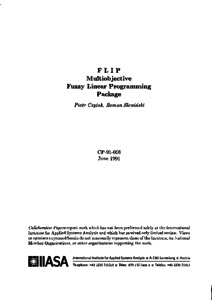# FLIP - Multiobjective Fuzzy Linear Programming Package

Czyzak, P. & Slowinski, R. (1991). FLIP - Multiobjective Fuzzy Linear Programming Package. IIASA Collaborative Paper. IIASA, Laxenburg, Austria: CP-91-008Preview Text CP-91-008.pdf Download (1MB) | Preview

## Abstract

FLIP (Fuzzy LInear Programming) is a package designed to help in analysis of multiobjective linear programming (MOLP) problems in an uncertain environment. The uncertainty of data is modeled by L-R type fuzzy numbers. They can appear in the objective functions as well as on the both sides of the constraints.

The input data to the FLIP package include the characteristics of the analyzed fuzzy MOLP problem, i.e., the number of criteria, constraints and decision variables, fuzzy cost coefficients for every objective and fuzzy coefficients of LHS and RHS for all constraints. The data loading is supported by a graphical presentation of fuzzy coefficients. The calculation is preceded by a transformation of the fuzzy MOLP problem into a multiobjective linear fractional program. It is then solved with an interactive method using a linear programming procedure as the only optimiser. In every iteration, one gets a series of solutions that are presented very clearly in a graphical and numerical form.

In FLIP, interaction with the user takes place at two levels: first, when safety parameters have to be defined in the transformation phase, and second, when the associate deterministic problem is solved.

The package is written in TURBO-Pascal and can be used on microcomputers compatible with IBM-PC XT/AT with hard disc and a graphic card.

Item Type: Monograph (IIASA Collaborative Paper) Methodology of Decision Analysis (MDA) IIASA Import 15 Jan 2016 02:01 27 Aug 2021 17:14 https://pure.iiasa.ac.at/3572View Item